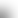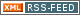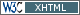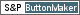www.LinuxHowtos.org howtos, tips&tricks and tutorials for linuxfrom small one page howto to huge articles all in one place

search text in:

Poll
Which screen resolution do you use?

poll results

using iotop to find disk usage hogs

using iotop to find disk usage hogs

words:

887

views:

155133

userrating:

average rating: 1.8 (88 votes) (1=very good 6=terrible)

May 25th. 2007:
Words

486

Views

246522

Workaround and fixes for the current Core Dump Handling vulnerability affected kernels

Workaround and fixes for the current Core Dump Handling vulnerability affected kernels

words:

161

views:

129725

userrating:

average rating: 1.3 (28 votes) (1=very good 6=terrible)

April, 26th. 2006:

You are here: manpages

math.h

Section: POSIX Programmer's Manual (0P)
Updated: 2013

PROLOG

This manual page is part of the POSIX Programmer's Manual. The Linux implementation of this interface may differ (consult the corresponding Linux manual page for details of Linux behavior), or the interface may not be implemented on Linux.

delim \$\$

NAME

math.h --- mathematical declarations

SYNOPSIS

#include <math.h>

DESCRIPTION

Some of the functionality described on this reference page extends the ISO C standard. Applications shall define the appropriate feature test macro (see the System Interfaces volume of POSIX.1-2008, Section 2.2, The Compilation Environment) to enable the visibility of these symbols in this header. The <math.h> header shall define at least the following types:
float_t
A real-floating type at least as wide as float.
double_t
A real-floating type at least as wide as double, and at least as wide as float_t. If FLT_EVAL_METHOD equals 0, float_t and double_t shall be float and double, respectively; if FLT_EVAL_METHOD equals 1, they shall both be double; if FLT_EVAL_METHOD equals 2, they shall both be long double; for other values of FLT_EVAL_METHOD, they are otherwise implementation-defined. The <math.h> header shall define the following macros, where real-floating indicates that the argument shall be an expression of real-floating type:

int fpclassify(real-floating x);
int isfinite(real-floating x);
int isgreater(real-floating x, real-floating y);
int isgreaterequal(real-floating x, real-floating y);
int isinf(real-floating x);
int isless(real-floating x, real-floating y);
int islessequal(real-floating x, real-floating y);
int islessgreater(real-floating x, real-floating y);
int isnan(real-floating x);
int isnormal(real-floating x);
int isunordered(real-floating x, real-floating y);
int signbit(real-floating x);
The <math.h> header shall define the following symbolic constants. The values shall have type double and shall be accurate within the precision of the double type.
M_E
Value of \$e\$
M_LOG2E
Value of \$log_ 2" " e\$
M_LOG10E
Value of \$log_ 10" " e\$
M_LN2
Value of \$log_ e" " 2\$
M_LN10
Value of \$log_ e" " 10\$
M_PI
Value of \$pi\$
M_PI_2
Value of \$pi /2\$
M_PI_4
Value of \$pi /4\$
M_1_PI
Value of \$1/ pi\$
M_2_PI
Value of \$2/ pi\$
M_2_SQRTPI
Value of \$2/ sqrt pi\$
M_SQRT2
Value of \$sqrt 2\$
M_SQRT1_2
Value of \$1/ sqrt 2\$ The <math.h> header shall define the following symbolic constant:
MAXFLOAT
Same value as FLT_MAX in <float.h>. The <math.h> header shall define the following macros:
HUGE_VAL
A positive double constant expression, not necessarily representable as a float. Used as an error value returned by the mathematics library. HUGE_VAL evaluates to +infinity on systems supporting IEEE Std 754-1985.
HUGE_VALF
A positive float constant expression. Used as an error value returned by the mathematics library. HUGE_VALF evaluates to +infinity on systems supporting IEEE Std 754-1985.
HUGE_VALL
A positive long double constant expression. Used as an error value returned by the mathematics library. HUGE_VALL evaluates to +infinity on systems supporting IEEE Std 754-1985.
INFINITY
A constant expression of type float representing positive or unsigned infinity, if available; else a positive constant of type float that overflows at translation time.
NAN
A constant expression of type float representing a quiet NaN. This macro is only defined if the implementation supports quiet NaNs for the float type. The following macros shall be defined for number classification. They represent the mutually-exclusive kinds of floating-point values. They expand to integer constant expressions with distinct values. Additional implementation-defined floating-point classifications, with macro definitions beginning with FP_ and an uppercase letter, may also be specified by the implementation.

FP_INFINITE FP_NAN FP_NORMAL FP_SUBNORMAL FP_ZERO
The following optional macros indicate whether the fma() family of functions are fast compared with direct code:

FP_FAST_FMA FP_FAST_FMAF FP_FAST_FMAL
If defined, the FP_FAST_FMA macro shall expand to the integer constant 1 and shall indicate that the fma() function generally executes about as fast as, or faster than, a multiply and an add of double operands. If undefined, the speed of execution is unspecified. The other macros have the equivalent meaning for the float and long double versions. The following macros shall expand to integer constant expressions whose values are returned by ilogb(x) if x is zero or NaN, respectively. The value of FP_ILOGB0 shall be either {INT_MIN} or -{INT_MAX}. The value of FP_ILOGBNAN shall be either {INT_MAX} or {INT_MIN}.

FP_ILOGB0 FP_ILOGBNAN
The following macros shall expand to the integer constants 1 and 2, respectively;

MATH_ERRNO MATH_ERREXCEPT
The following macro shall expand to an expression that has type int and the value MATH_ERRNO, MATH_ERREXCEPT, or the bitwise-inclusive OR of both:

math_errhandling
The value of math_errhandling is constant for the duration of the program. It is unspecified whether math_errhandling is a macro or an identifier with external linkage. If a macro definition is suppressed or a program defines an identifier with the name math_errhandling , the behavior is undefined. If the expression (math_errhandling & MATH_ERREXCEPT) can be non-zero, the implementation shall define the macros FE_DIVBYZERO, FE_INVALID, and FE_OVERFLOW in <fenv.h>. The following shall be declared as functions and may also be defined as macros. Function prototypes shall be provided.

double      acos(double);
float       acosf(float);
double      acosh(double);
float       acoshf(float);
long double acoshl(long double);
long double acosl(long double);
double      asin(double);
float       asinf(float);
double      asinh(double);
float       asinhf(float);
long double asinhl(long double);
long double asinl(long double);
double      atan(double);
double      atan2(double, double);
float       atan2f(float, float);
long double atan2l(long double, long double);
float       atanf(float);
double      atanh(double);
float       atanhf(float);
long double atanhl(long double);
long double atanl(long double);
double      cbrt(double);
float       cbrtf(float);
long double cbrtl(long double);
double      ceil(double);
float       ceilf(float);
long double ceill(long double);
double      copysign(double, double);
float       copysignf(float, float);
long double copysignl(long double, long double);
double      cos(double);
float       cosf(float);
double      cosh(double);
float       coshf(float);
long double coshl(long double);
long double cosl(long double);
double      erf(double);
double      erfc(double);
float       erfcf(float);
long double erfcl(long double);
float       erff(float);
long double erfl(long double);
double      exp(double);
double      exp2(double);
float       exp2f(float);
long double exp2l(long double);
float       expf(float);
long double expl(long double);
double      expm1(double);
float       expm1f(float);
long double expm1l(long double);
double      fabs(double);
float       fabsf(float);
long double fabsl(long double);
double      fdim(double, double);
float       fdimf(float, float);
long double fdiml(long double, long double);
double      floor(double);
float       floorf(float);
long double floorl(long double);
double      fma(double, double, double);
float       fmaf(float, float, float);
long double fmal(long double, long double, long double);
double      fmax(double, double);
float       fmaxf(float, float);
long double fmaxl(long double, long double);
double      fmin(double, double);
float       fminf(float, float);
long double fminl(long double, long double);
double      fmod(double, double);
float       fmodf(float, float);
long double fmodl(long double, long double);
double      frexp(double, int *);
float       frexpf(float, int *);
long double frexpl(long double, int *);
double      hypot(double, double);
float       hypotf(float, float);
long double hypotl(long double, long double);
int         ilogb(double);
int         ilogbf(float);
int         ilogbl(long double);
double      j0(double);
double      j1(double);
double      jn(int, double);
double      ldexp(double, int);
float       ldexpf(float, int);
long double ldexpl(long double, int);
double      lgamma(double);
float       lgammaf(float);
long double lgammal(long double);
long long   llrint(double);
long long   llrintf(float);
long long   llrintl(long double);
long long   llround(double);
long long   llroundf(float);
long long   llroundl(long double);
double      log(double);
double      log10(double);
float       log10f(float);
long double log10l(long double);
double      log1p(double);
float       log1pf(float);
long double log1pl(long double);
double      log2(double);
float       log2f(float);
long double log2l(long double);
double      logb(double);
float       logbf(float);
long double logbl(long double);
float       logf(float);
long double logl(long double);
long        lrint(double);
long        lrintf(float);
long        lrintl(long double);
long        lround(double);
long        lroundf(float);
long        lroundl(long double);
double      modf(double, double *);
float       modff(float, float *);
long double modfl(long double, long double *);
double      nan(const char *);
float       nanf(const char *);
long double nanl(const char *);
double      nearbyint(double);
float       nearbyintf(float);
long double nearbyintl(long double);
double      nextafter(double, double);
float       nextafterf(float, float);
long double nextafterl(long double, long double);
double      nexttoward(double, long double);
float       nexttowardf(float, long double);
long double nexttowardl(long double, long double);
double      pow(double, double);
float       powf(float, float);
long double powl(long double, long double);
double      remainder(double, double);
float       remainderf(float, float);
long double remainderl(long double, long double);
double      remquo(double, double, int *);
float       remquof(float, float, int *);
long double remquol(long double, long double, int *);
double      rint(double);
float       rintf(float);
long double rintl(long double);
double      round(double);
float       roundf(float);
long double roundl(long double);
double      scalbln(double, long);
float       scalblnf(float, long);
long double scalblnl(long double, long);
double      scalbn(double, int);
float       scalbnf(float, int);
long double scalbnl(long double, int);
double      sin(double);
float       sinf(float);
double      sinh(double);
float       sinhf(float);
long double sinhl(long double);
long double sinl(long double);
double      sqrt(double);
float       sqrtf(float);
long double sqrtl(long double);
double      tan(double);
float       tanf(float);
double      tanh(double);
float       tanhf(float);
long double tanhl(long double);
long double tanl(long double);
double      tgamma(double);
float       tgammaf(float);
long double tgammal(long double);
double      trunc(double);
float       truncf(float);
long double truncl(long double);
double      y0(double);
double      y1(double);
double      yn(int, double);
The following external variable shall be defined:

extern int signgam;
The behavior of each of the functions defined in <math.h> is specified in the System Interfaces volume of POSIX.1-2008 for all representable values of its input arguments, except where stated otherwise. Each function shall execute as if it were a single operation without generating any externally visible exceptional conditions.

The following sections are informative.

APPLICATION USAGE

The FP_CONTRACT pragma can be used to allow (if the state is on) or disallow (if the state is off) the implementation to contract expressions. Each pragma can occur either outside external declarations or preceding all explicit declarations and statements inside a compound statement. When outside external declarations, the pragma takes effect from its occurrence until another FP_CONTRACT pragma is encountered, or until the end of the translation unit. When inside a compound statement, the pragma takes effect from its occurrence until another FP_CONTRACT pragma is encountered (including within a nested compound statement), or until the end of the compound statement; at the end of a compound statement the state for the pragma is restored to its condition just before the compound statement. If this pragma is used in any other context, the behavior is undefined. The default state (on or off) for the pragma is implementation-defined. Applications should use FLT_MAX as described in the <float.h> header instead of the obsolescent MAXFLOAT.

RATIONALE

Before the ISO/IEC 9899:1999 standard, the math library was defined only for the floating type double. All the names formed by appending 'f' or 'l' to a name in <math.h> were reserved to allow for the definition of float and long double libraries; and the ISO/IEC 9899:1999 standard provides for all three versions of math functions. The functions ecvt(), fcvt(), and gcvt() have been dropped from the ISO C standard since their capability is available through sprintf().

FUTURE DIRECTIONS

None.

<float.h>, <stddef.h>, <sys_types.h> The System Interfaces volume of POSIX.1-2008, Section 2.2, The Compilation Environment, acos(), acosh(), asin(), asinh(), atan(), atan2(), atanh(), cbrt(), ceil(), copysign(), cos(), cosh(), erf(), erfc(), exp(), exp2(), expm1(), fabs(), fdim(), floor(), fma(), fmax(), fmin(), fmod(), fpclassify(), frexp(), hypot(), ilogb(), isfinite(), isgreater(), isgreaterequal(), isinf(), isless(), islessequal(), islessgreater(), isnan(), isnormal(), isunordered(), j0(), ldexp(), lgamma(), llrint(), llround(), log(), log10(), log1p(), log2(), logb(), lrint(), lround(), modf(), nan(), nearbyint(), nextafter(), pow(), remainder(), remquo(), rint(), round(), scalbln(), signbit(), sin(), sinh(), sqrt(), tan(), tanh(), tgamma(), trunc(), y0()

Portions of this text are reprinted and reproduced in electronic form from IEEE Std 1003.1, 2013 Edition, Standard for Information Technology -- Portable Operating System Interface (POSIX), The Open Group Base Specifications Issue 7, Copyright (C) 2013 by the Institute of Electrical and Electronics Engineers, Inc and The Open Group. (This is POSIX.1-2008 with the 2013 Technical Corrigendum 1 applied.) In the event of any discrepancy between this version and the original IEEE and The Open Group Standard, the original IEEE and The Open Group Standard is the referee document. The original Standard can be obtained online at http://www.unix.org/online.html .

Any typographical or formatting errors that appear in this page are most likely to have been introduced during the conversion of the source files to man page format. To report such errors, see https://www.kernel.org/doc/man-pages/reporting_bugs.html .

Index

PROLOG
NAME
SYNOPSIS
DESCRIPTION
APPLICATION USAGE
RATIONALE
FUTURE DIRECTIONS||- Powered by- Running on-
Copyright 2004-2020 Sascha Nitsch Unternehmensberatung GmbH::- Level Triple-A Conformance to Web Content Accessibility Guidelines 1.0 -
- Copyright and legal notices -